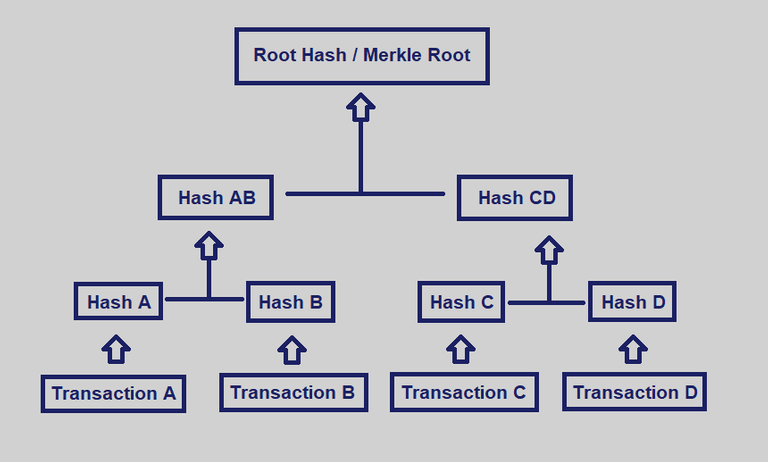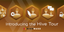# [Blockchain for Geeks - 1] The Merkle Tree/Root

in #hivelast year

As a geek myself and in the same way that I tried a new concept on my @imtase account with my post on `Unboxing/Test: Micro Wireless Saramonic`, I would like to try a new series of post on the blockchain (along a personal project) and this is the first episode 😊

### Merkle Tree & Merkle Root

Merkle trees (also known as binary hash trees) are used to encode blockchain data in a more efficient and secure way.

A block in a blockchain contain a number of transactions (ex: https://hiveblocks.com/b/51167279), each transaction of the block (the first line of the picture below) is hashed. This means that it creates a fixed-length string of numbers and letters from the transaction (the second line of the picture below) that serves as an index for a hash table (cf. wikipedia).

Then each pair of transactions (the third line of the picture below) is concatenated and hashed together, the operation is repeated until a single hash is obtained for the whole block. The last hash is called Merkle Root.

In case of an odd number of transactions, one transaction is doubled and its hash is concatenated with itself.

This process create a tree-like structure such that each hash is linked to its parent following a parent-child tree-like relation.

The Merkle Root is stored in the header of the block while the transaction hash is stored in the transaction.The advantage of this method is to be able to validate that all the transactions present in the block are genuine or to verify a transaction that claims to come from a specific block.

### Hive-Engine case studies

On Hive-Engine the function to calculate the Merkle Root look like this

``````  // calculate the Merkle root of the block ((#TA + #TB) + (#TC + #TD) )
calculateMerkleRoot(transactions) {
if (transactions.length <= 0) return '';

const tmpTransactions = transactions.slice(0, transactions.length);
const newTransactions = [];
const nbTransactions = tmpTransactions.length;

for (let index = 0; index < nbTransactions; index += 2) {
const left = tmpTransactions[index].hash;
const right = index + 1 < nbTransactions ? tmpTransactions[index + 1].hash : left;

const leftDbHash = tmpTransactions[index].databaseHash;
const rightDbHash = index + 1 < nbTransactions
? tmpTransactions[index + 1].databaseHash
: leftDbHash;

newTransactions.push({
hash: SHA256(left + right).toString(enchex),
databaseHash: SHA256(leftDbHash + rightDbHash).toString(enchex),
});
}

if (newTransactions.length === 1) {
return {
hash: newTransactions.hash,
databaseHash: newTransactions.databaseHash,
};
}

return this.calculateMerkleRoot(newTransactions);
}
``````

https://github.com/hive-engine/steemsmartcontracts/blob/hive-engine/libs/Block.js

##### Which can be decomposed as follows

1/ We send an array of transactions to the function
2/ If no transaction the function return nothing (no hash)
3/ to preserve the original data the function copy the array of transactions in `tmpTransactions`
4/ the function create an array `newTransactions` to repeat the operation until we get only one hash
5/ the function get the number of transactions and put it in `nbTransactions`
6/ for each pair of transactions
6.1 the left hash = the 1st transaction of the pair
6.2 the right hash = the 2nd transaction of the pair (if not have use the 1st transaction of the pair)
6.3 Create a new hash with (left + right) and put it in `newTransactions`
7/ if `newTransactions` contain only one hash we stop and return the hash
8/ `newTransactions` contain more than one hash the function is executed again

### HIVE

On HIVE blockchain the function to calculate the Merkle Root look like this

``````  checksum_type signed_block::calculate_merkle_root()const
{
if( transactions.size() == 0 )
return checksum_type();

vector<digest_type> ids;
ids.resize( transactions.size() );
for( uint32_t i = 0; i < transactions.size(); ++i )
ids[i] = transactions[i].merkle_digest();

vector<digest_type>::size_type current_number_of_hashes = ids.size();
while( current_number_of_hashes > 1 )
{
// hash ID's in pairs
uint32_t i_max = current_number_of_hashes - (current_number_of_hashes&1);
uint32_t k = 0;

for( uint32_t i = 0; i < i_max; i += 2 )
ids[k++] = digest_type::hash( std::make_pair( ids[i], ids[i+1] ) );

if( current_number_of_hashes&1 )
ids[k++] = ids[i_max];
current_number_of_hashes = k;
}
return checksum_type::hash( ids );
}

} } // hive::protocol
``````

And if I understood it correctly the hash function uses an implementation of the FNV-1a Fowler–Noll–Vo function (wikipedia)

``````  /** FNV-1a implementation https://en.wikipedia.org/wiki/Fowler-Noll-Vo_hash_function#FNV-1a_hash */
struct hasher {
static int32_t hash( uint8_t byte, uint32_t _hash )
{
return ( byte ^ _hash ) * prime;
}

static uint32_t hash( uint32_t four_bytes, uint32_t _hash = offset_basis )
{
uint8_t* ptr = (uint8_t*) &four_bytes;
_hash = hash( *ptr++, _hash );
_hash = hash( *ptr++, _hash );
_hash = hash( *ptr++, _hash );
return  hash( *ptr,   _hash );
}

private:
// Recommended by http://isthe.com/chongo/tech/comp/fnv/
static const uint32_t prime         = 0x01000193; //   16777619
static const uint32_t offset_basis  = 0x811C9DC5; // 2166136261
};
``````

sources:If you like my work, consider voting for my witness
it will only cost you 30 seconds of your time 😉

My witness presentation: @mintrawa a Gen X - Geek 🤓 Gamer 🎮 Traveler ⛩️ WitnessYou received more than 300 upvotes.Your next target is to reach 400 upvotes.
If you no longer want to receive notifications, reply to this comment with the word `STOP`Time to go on your Hive Tour